## Tamilnadu Samacheer Kalvi 9th Maths Solutions Chapter 7 Mensuration Ex 7.3

Question 1.
Find the volume of a cuboid whose dimensions are
(i) length = 12 cm, breadth = 8 cm, height = 6 cm
(ii) length = 60 m, breadth = 25 m, height = 1.5 m
Solution:
(i) l = 12 cm
b = 8 cm
h = 6 cm
Volume of the cuboid = lbh
= 12 × 8 × 6 cm3 = 576 cm3

(ii) l = 60 m
b = 25 m
h = 1.5 m
Volume of the cuboid = lbh = 60 × 25 × 1.5m3 = 2250 m3Question 2.
The dimensions of a match box are 6 cm × 3.5 cm × 2.5 cm. Find the volume of a packet containing 12 such match boxes.
Solution:
Dimensions of a match box = 6 cm × 3.5 cm × 2.5 cm
V = (6 × 3.5 × 2.5) cm3 = 52.5 cm3
Volume of 12 such boxes = 12 × 52.5 cm3 = 630 cm3

Question 3.
The length, breadth and height of a chocolate box are in the ratio 5 : 4 : 3. If its volume is 7500 cm3, then find its dimensions.
Solution:
Let 1 ratio = x then 5 : 4 : 3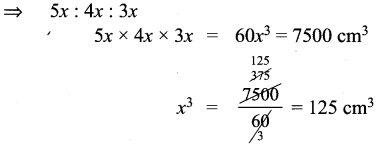x = 5 cm
∴ 5x = 5 × 5 = 25 cm
4x = 4 × 5 = 20 cm
3x = 3 × 5 = 15 cm
∴ Dimensions of a chocolate box are 25 cm × 20 cm × 15 cm

Question 4.
The length, breadth and depth of a pond are 20.5 m, 16 m and 8 m respectively. Find the capacity of the pond in litres.
Solution:
l = 20.5 m
b = 16 m
h = 8 m
∴ Capacity = Volume = lb h = (20.5 × 16 × 8) m3 = 2624 m3
1 m3 = 1000 litres
∴ 2624 m3 = 2624000 litresQuestion 5.
The dimensions of a brick are 24 cm × 12 cm × 8 cm. How many such bricks will be required to build a wall of 20 m length, 48 cm breadth and 6 m height?
Solution:
l = 24 cm
b = 12 cm
h = 8 cm
Volume of the brick = lbh = (24 × 12 × 8) cm3 = 2304 cm3
Wall dimensions are :
L = 20 m = 2000 cm
B = 48 cm
H = 6 m = 600 cm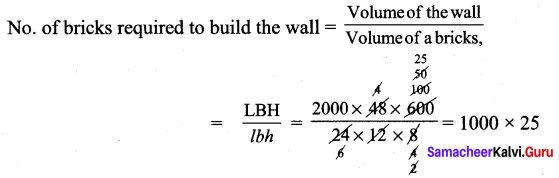No. of bricks required = 25000

Question 6.
The volume of container is 1440 m3. The length and breadth of the container are 15 m and 8 m respectively. Find its height.
Solution:
V = l × b × h = 1440 m3
15 × 8 × h = 1440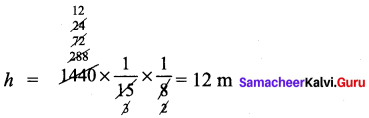Question 7.
Find the volume of a cube each of whose side is
(i) 5 cm
(ii) 3.5 m
(iii) 21 cm
Solution:
(i) side of a cube (a) = 5 cm
Volume of a cube V = a3 = 5 × 5 × 5 = 125 cm3

(ii) a = 3.5 m
V = a3 = 3.5 × 3.5 × 3.5 m3 = 42.875 m3

(iii) a = 21 cm 23 = 21 × 21 × 21 cm3 = 9261 cm3

Question 8.
A cubical milk tank holds 125000 litres of milk. Find the length of its side in metres.
Solution:
V = a3 = 125000 litres = 125 m3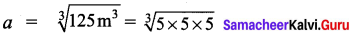∴ length of its side = 5 m.

Question 9.
A metallic cube with side 15 cm is melted and formed into a cuboid. If the length and height of the cuboid is 25 cm and 9 cm respectively then find the breadth of the cuboid.
Solution:
The volume of the cuboid formed = The volume of the cube melted.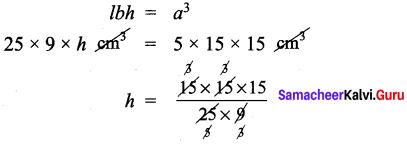height of the cuboid = 15 cm